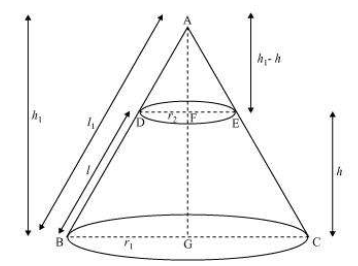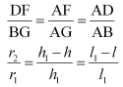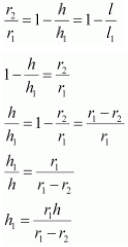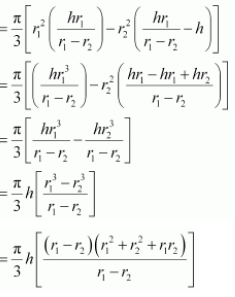Newbie

# Derive the formula for the volume of the frustum of a cone. Q.7

• 0

Find the important question of ncert class 10th surface areas and volumes of exercise 13.5 question number 7 . Give me the simplest and easiest form of this question and also find the best solution of this question.

Share

1. Consider the same diagram as the previous question.Now, approach the question in the same way as the previous one and prove that

Again,Now, rearrange them in terms of h and h1The total volume of frustum of the cone will be = Volume of cone ABC – Volume of cone ADE

= (⅓)πr12h1 -(⅓)πr22(h1 – h)

= (π/3)[r12h1-r22(h1 – h)]Now, solving this we get,

∴ Volume of frustum of the cone = (⅓)πh(r12+r22+r1r2)

Chapter 13, Surface Areas and Volumes, of class 10 Maths, is one of the most important chapters. The weightage of this chapter in the final exam is around 12 to 13 marks. On average, there are 4 questions asked from this chapter, based on surface areas and volumes. The distribution of marks with respect to questions are 3+3+3+4, where marks could vary depending upon the question. Topics covered in Chapter 13, Surface Areas and Volumes are;

• The surface area of a combination of Solids
• The volume of a combination of solids
• Conversion of solid from one shape to another
• Frustum of a cone
• 0